Salinas, C., Ananías, R., and Vasco, D. (2018). "Modelling of radio-frequency heating of piles of Pinus radiata wood," BioRes. 13(1), 945-953.

#### Abstract

The present work studied the numerical modelling of heat transfer in a pile of Pinus radiata samples of a square cross-section by using radio frequency heating.  More precisely, the study focused on the effects of the energy transferred to a dielectric material (wood) from an electromagnetic field, which required the calculation of the dielectric loss factor and its correlation with conservative equations. In this way, the temperature distribution across Pinus radiata samples was obtained through the integration of the energy equation using the finite volume method. The numerical results were compared to experimental data obtained from three experiments of radio-frequency heating of wood samples of a cross-sectional area of 4 in × 4 in, 3.1-m-long, and 20 cm of separation between plates. According to the observed linear behavior of the heat transfer process, the numerical results of the transient variation of temperature were in agreement with the experimental data.

Carlos Salinas,a,* Ruben A. Ananias,b and Diego A. Vasco c

The present work studied the numerical modelling of heat transfer in a pile of Pinus radiata samples of a square cross-section by using radio frequency heating. More precisely, the study focused on the effects of the energy transferred to a dielectric material (wood) from an electromagnetic field, which required the calculation of the dielectric loss factor and its correlation with conservative equations. In this way, the temperature distribution across Pinus radiata samples was obtained through the integration of the energy equation using the finite volume method. The numerical results were compared to experimental data obtained from three experiments of radio-frequency heating of wood samples of a cross-sectional area of 4 in × 4 in, 3.1-m-long, and 20 cm of separation between plates. According to the observed linear behavior of the heat transfer process, the numerical results of the transient variation of temperature were in agreement with the experimental data.

Keywords: Dielectric heating; Dielectric loss factor; Heat treatment; Pinus radiata; Temperature simulation

Contact information: a: Grupo de Investigación en Tecnologías del Secado y Tratamientos Térmicos de la Madera, Departamento de Ingeniería Mecánica, Universidad del Bío-Bío, Concepción, Chile; b: Grupo de Investigación en Tecnologías del Secado y Tratamientos Térmicos de la Madera, Departamento de Ingeniería en Maderas, Universidad del Bío-Bío, Concepción, Chile; c: Departamento de Ingeniería Mecanica, Universidad de Santiago de Chile, Santiago, Chile; *Corresponding author: casali@ubiobio.cl

INTRODUCTION

Pinus radiata D. Don is one of the most important wood species in the Chilean wood industry (Infor 2016). P. radiata is often used as packing material, which requires it to be sterilized through vapour to fulfill phytosanitary requirements (Ananías et al. 2013). One alternative to using vapour to sterilize piles of Pinus radiata wood is to heat it through radio frequency electromagnetic radiation (Esquivel-Reyes et al. 2017). In contrast, dielectric heating, which implies the implementation of technologies such as radio frequency and microwaves, is used in several industries related to sterilization and drying of inert and organic materials (Wang and Wang 2008).

In traditional heating and drying processes, the thermal resistance of wood logs and woodpiles limits heat and mass transfer. Therefore, the slowest heating or drying regions are found in the thermal center of the treated product, which leads to over-processing of surface regions. However, radio-frequency heating is dependent upon the product itself, its geometry, and its thermophysical properties. As radio-frequency electromagnetic waves heat volumetrically, they can theoretically improve heating uniformity (Misra et al. 2015).

The heating process of wood for sterilization implies the loss of moisture to a lesser degree. Nevertheless, this phenomena could be important, depending on the performed study (Huang et al. 2013). To perform the modelling of the sterilization process of wood, both the heat and mass transfers must be considered (Jia et al. 2017).

Radio-frequency radiation consists of electromagnetic waves and represents a nonionizing type of radiation that causes molecular motion through migration of ions and rotation of dipoles without altering the molecular structure. A material can be heated by applying energy to it in the form of electromagnetic waves (Orfeuil 1987). The heating effect originates from the ability of an electric field to exert a force on charged particles. The heating effect depends on the frequency and the applied power of the electromagnetic waves, as well as on the material properties.

The dielectric properties of materials are defined by two parameters, the dielectric constant and the dielectric loss factor. The dielectric constant describes the ability of a molecule to be polarized by an electric field. The dielectric loss factor measures the efficiency with which the energy of the electromagnetic radiation is converted into heat. The ratio of the dielectric loss factor to its dielectric constant is expressed as the dissipation factor (Haswell and Kingston 1997). While electromagnetic waves penetrate the sample, energy is absorbed depending on the dissipation factor of the material. The greater the dissipation factor of a sample, the less electromagnetic energy will penetrate into it because it is rapidly absorbed and dissipated. Radio-frequency electromagnetic energy is transformed into heat via two mechanisms that occur simultaneously: ionic conduction and dipole rotation. The dissipation factor changes with temperature, which affects ion mobility and concentration. The second mechanism (dipole rotation) refers to the alignment of molecules that have a permanent or induced dipole moment. The relative contribution of each energy conversion mechanism depends mainly on the temperature. For small molecules, such as water, the contribution of a dipole rotation decreases as the sample temperature increases, whereas the contribution of ionic conduction increases as the product temperature is increased. Energy delivery to the biomaterial by radio-frequency radiation depends on a number of variables such as power input, exposure time, material properties, and the size of the sample. However, the greater the dissipation factor of a sample, the less it will be penetrated by the electromagnetic wave. Hence, in large samples with high dissipation factors, heating of the central part may still depend on thermal conductance (Misra et al. 2015).

From the modelling point of view, two approaches are found. The first one is focused on the effects of the electromagnetic field on the heat transfer to wood, whose dielectric parameters are known, particularly the dielectric loss factor, which are correlated through conservation equations (Koumoutsakos et al. 2001; Jia et al. 2015). The second approach is based on the description of the electric and magnetic fields according to the Maxwell equations (Bucki and Perré 2003, Marra et al. 2009). The present study used the former approach. Therefore, the dielectric loss factor was understood as the wood capacity as dielectric material of transforming electrical energy into thermal energy (Resch 2006; Avramidis 2016).

Particularly, a methodology for determining the dielectric loss factor from heating curves is presented, which is useful to determine the required power of the energy source in a differential mathematical model. The main goal of the present work is the numerical calculation of the spatial and transient distribution of temperature during the sterilization of moist P. radiata D. Don wood by using radio-frequency heating.

EXPERIMENTAL

Materials

The radio-frequency (RF) heating process was performed in triplicate on wood piles of square section samples measuring 4 in × 4 in and 3.1-m-long (total volume of 3 m3). The Pinus radiata D. Don wood used was 14-years-old and obtained from the Bío Bío region in Chile. The samples of green wood were collected from the inner wood of randomly selected logs.

The separation between plates was kept at 200 mm. The wood load was mounted in the RF equipment (Saga HF-VD30SA, Shijiazhuang, Hebei, China), as shown in Fig. 1. The work power and the oscillation frequency were set at 30 kW and 6.78 MHz, respectively.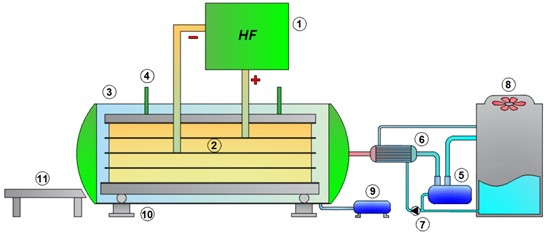Fig. 1. Radio-frequency equipment: 1) High frequency (HF) generator, 2) Plate electrodes, 3) Autoclave, 4) Hydraulic system, 5) Vacuum pump, 6) Cooling tank, 7) Water pump, 8) Cooling tower, 9) Condensate tank, 10) Load cells, and 11) Trolley (Torres 2017)

Temperatures during heating were collected every 10 s using a data acquisition equipment and optical fiber temperature sensors (Oriental Rayzer, ORZ-FTM1000, Beijing, China) distributed as is shown in Fig. 2.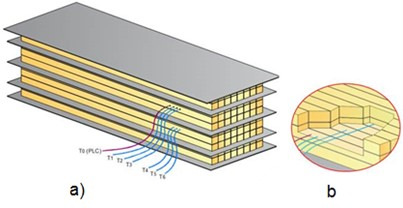Fig. 2. Wood load (4 in × 4 in × 3.1 m): a) position of temperature sensors between plates (20 cm), and b) detail of the position of the sensors inside the wood load

Methods

Phenomenological modelling

The first implemented mathematical model was found in the adsorbed thermal energy due to the presence of an electromagnetic field, shown in Eq. 1,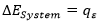(1)

which describes an energy balance between the heat flow generated by RF ( and the variation of the energy of the system (. If a transformation between electromagnetic energy and sensible thermal energy is considered (dielectric heating) without heat loss, and the inner conductive thermal resistance is considered to be low, the following ordinary differential equation that describes the transient variation of the average temperature of wood is obtained (Wang et al. 2005) in Eq. 2,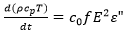(2)

where cp is the specific heat (J/kg°C), is the apparent density of wood (kg/m3), E is the intensity of the electric field (V/m), f is the frequency (Hz), is the dielectric loss factor, and is a constant equal to 55.33 × 10-12. In this study, saturated wood with a homogeneous humidity content was considered, and the loss of humidity was not taken into account, therefore the differential equation may be written as,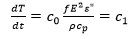(3)

which may be solved analytically using Eqs. 4 and 5,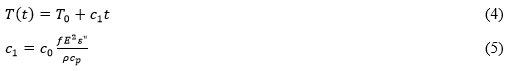which represents a linear increasing of temperature as a function of time.

RESULTS AND DISCUSSION

The experimental results for the transient variation of temperature obtained during the dielectric heating of wood are shown in Fig. 2.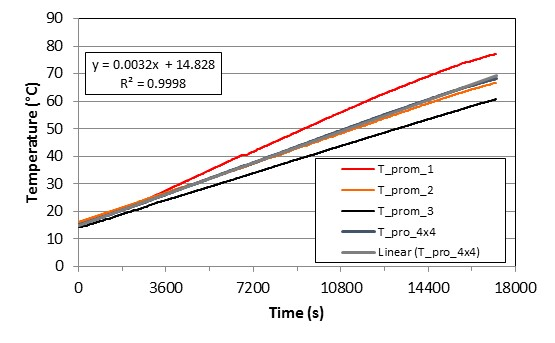Fig. 2. Average values of temperature registered during the radio-frequency heating (24 kW) of wood piles of samples of square cross-section (4 in × 4 in and 3.1-m-long, total volume of 3 m3)

The results shown in Fig. 2 clearly demonstrate that there was a linear increase in the average temperature of the wood in all tests. Moreover, it is important to note that the average temperature of all tests (T_pro_4x4) had a linear coefficient of determination almost equal to one (R= 0.9998). These experimental results validate the mathematical model represented by Eq. 2, which solution is given by Eq. 4. This solution is valid when there is no heat losses and the inner thermal resistance is negligible. Consequently, and according to Zhou and Avramidis (1999), the average effective power transferred to the wood load is given by Eq. 6,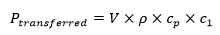(6)

Then, considering the values for density and specific heat of P. radiata of 800 kg/mand 2.2 J/kg°C, respectively (Acuña 2017), and the value calculated from Eq. 5, the following result is obtained in Eq. 7: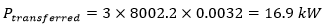(7)

Finally, if the transferred power and the dielectric field parameters (f and E) are known, the dielectric loss factor can be obtained through Eq. 8: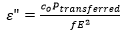(8)

One-dimensional Model

A second approach is based on the description of the spatial distribution of temperature. Therefore, mathematical models based on differential transport equations that describe the variation of the dependent variable in a one-dimensional (1D), two-dimensional (2D), or three-dimensional (3D) space is required. The available temperature data were collected along the horizontal symmetry axis, located in the cross-sectional plane in three characteristic points: at the surface, middle, and center of the wood load. Therefore, a one-dimensional heating model was considered using the previously obtained values of the transferred power () and the dielectric loss factor (), when the inner thermal resistance was not considered. Under these considerations, the thermal energy balance of wood is given by Eq. 9,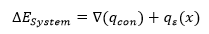(9)

where the equation represents an energy balance that considers the conductive heat transfer flow (W/m2), a generation term in function of the independent variable (x), which corresponds to the gained heat due to the electric field, both terms induce variation of the energy rate of the system . The generation term, a product of the transformation of electromagnetic energy in sensible thermal energy (dielectric heating), was added to the conductive heat transfer according to the Fourier’s Law, therefore Eq. 10 may be written as,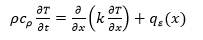(10)

where T is the temperature (°C), t is the time (s), x is the distance along the thickness of the wood pile (m), is the density (kg/m3), cp is the specific heat (J/kg ºC), k is the thermal conductivity (W/m ºC), and is the transferred heat from the electromagnetic field (W/m3). Equation 11 is under the following initial and boundary conditions,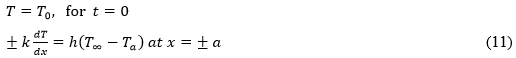where T0 is the initial temperture (°C), T is the environment temperature (°C), Tis the surface temperature of Wood (°C), and h is the convective coefficient (W/m2 ºC).

Before solving Eq. 10 according to the established conditions in Eq. 11, the values of h and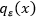are required. The determination of may be performed with the consideration that the energy from the wood to the inner side of the chamber of the radio-frequency equipment is equal to the transferred thermal energy from the inner side of the chamber to the environment (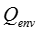). Therefore,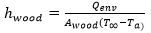(12)

where Awood is the surface area of wood (m2), as the ambient temperature (ºC), and,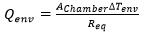(13)

where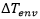is the variation of temperature (oC) between the inner and outer side of the chamber, A is the heat transfer area (m2), and Req is the equivalent thermal resistance (ºC/W), given by Eq. 14,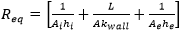(14)

where kwall is the thermal conductivity of the wall of the chamber of the radio-frequency equipment (W/m ºC), L is the thickness of the chamber (m), and subscripts (i) and (e) refer to the inner and outer side of the chamber, respectively. The term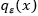is calculated according to the described preceding procedure for the temperature values close to the surface, the center, and the middle point between the center and the surface. From the first test (Fig. 3b), the obtained values of the temperature are shown in Fig. 3a.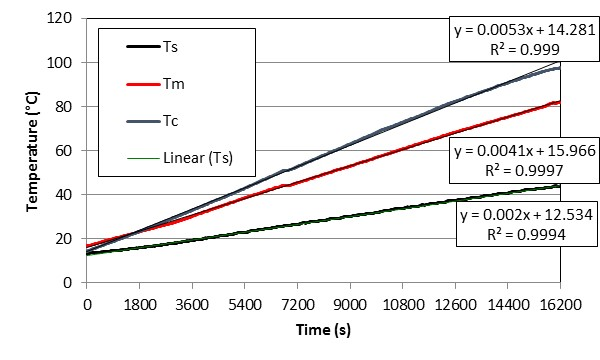a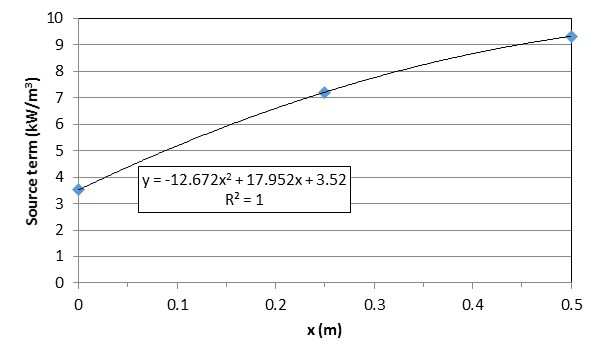b Fig. 3. Transient variation of temperature of the wood (a) and experimental values for the thermal energy source term according to the position (b).

After the numerical integration of Eq. 10 using the finite volume method, which is described by Salinas et al. 2015, the temperature distribution shown in Fig. 4 was obtained.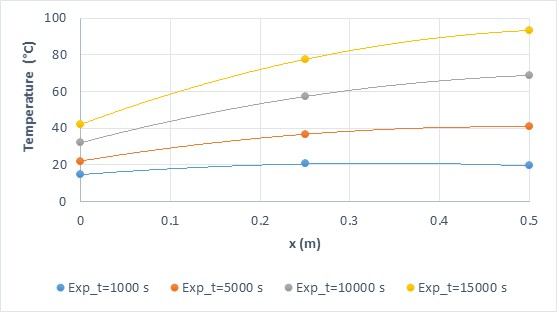Fig. 4. Spatial variation of temperatures obtained experimentally (discrete symbols) and numerically (segmented line): x = 0.025 m, and t = 10 s

The results showed a perfect correlation between the experimental and simulated data for x = 0.025 (m), and t = 10 (s). These numerical parameters were determined according to convergence analysis for numerical algorithms to solve the partial differential equations.

CONCLUSIONS

1. The measured spatial and transient variation of the average temperatures of a wood load during radio-frequency heating showed a linear behavior and therefore may be easily simulated by a linear mathematical model.
2. The variation of the temperature between the surface and the center followed the non-uniformity of the electric field and to the anisotropy of the dielectric material (wood).
3. The heating curves may be used for the determination of the dielectric properties, specifically the dielectric loss factor.
4. The spatial variation of the temperature may be modelled through the diffusive transport heat equation, considering a heat source that describes the transferred thermal energy from the electromagnetic field.
5. Given the linear behavior of the radio-frequency heating process, the simulated values of the transient variation of temperature were in agreement with the experimental values.

ACKNOWLEDGMENTS

This work was funded by the Conicyt Fondef-Idea 2-Etapas, project ID14I10231.

REFERENCES CITED

Acuña, F. (2017). Determinación Inversa de la Conductividad Térmica en Madera de Pinus radiata [Inverse Determination of the Thermal Conductivity of Pinus radiata Wood], Master’s Thesis, Departamento de Ingeniería en Maderas, Universidad del Bío-Bío, Concepción, Chile.

Ananías, R. A., Venegas, R., Salvo, L., and Elustondo, D. (2013). “Kiln schedule certification for industrial drying of radiata pine,” Wood. Fiber Sci. 45(1), 98-104.

Avramidis, S. (2016). “Dielectric properties of four softwood species at low-level radio

frequencies for optimized heating and drying,” Dry. Technol. 34(7), 753-760. DOI: 10.1080/07373937.2015.1072719

Bucki, M., and Perré, P. (2003). “Physical formulation and numerical modeling of high frequency heating of wood,” Dry. Technol. 21(7), 1151-1172. DOI: 10.1081/DRT-120023173

Esquivel-Reyes, H., Sepúlveda-Villarroel, V., Torres-Mella, J., Salvo-Sepúlveda, L., and Ananias, R. A. (2017). “Radio frequency heating times for sterilization radiata pine solid piles,” in: Proceeding of IRG48 Scientific Conference on Wood Protection, Ghent, Belgium, pp. 4-8.

Haswell, S. J., and Kingston, H. M. (1997). Microwave-Enhanced Chemistry: Fundamentals, American Chemical Society, Washington, D.C.

Huang, R., Wu, Y., Zhao, Y., Lu, J., Jiang, J., and Chen, Z. (2013). “Factors affecting the temperature increasing rate in wood during radio-frequency heating,” Dry. Technol. 31(2), 246-252. DOI: 10.1080/07373937.2012.728269

Infor. (2016). La Industria del Aserrío (Boletín Técnico No. 155) [The Industry of Sawmill (Technical Report No. 155), Instituto Forestal de Chile [Forestry Institute of Chile], Santiago de Chile, Chile.

Jia, X., Zhao, J., and Cai, Y. (2015). “Radio frequency vacuum drying of timber: Mathematical model and numerical analysis,” BioResources 10(3), 5440-5459. DOI: 10.15376/biores.10.3.5440-5459

Jia, X., Zhao, J., and Cai, Y. (2017). “Mass and heat transfer mechanism in wood during radio frequency/vacuum drying and numerical analysis,” J. Forestry Res. 28(1), 205-213. DOI: 10.1007/s11676-016-0269-3

Koumoutsakos, A., Avramidis, S., and Hatzikiriakos, S. (2001). “Radio frequency vacuum drying of wood. I. Mathematical model,” Dry. Technol. 19(1), 65-84. DOI: 10.1081/DRT-100001352

Marra, F., Zhang, L., and Lyng, J. G. (2009). “Radio frequency treatment of foods: Review of recent advances,” J. Food Eng. 91(4), 497-508. DOI: 10.1016/j.jfoodeng.2008.10.015

Misra, N. N., Cullen, P. J., Barba, F. J., Hii, L. H., Jaeger, H., Schmidt, J., Kovács, A., and Yoshida, H. (2015). “Emerging macroscopic pretreatment,” in: Food Waste Recovery – Processing Technologies and Industrial Techniques, Elsevier, San Diego, USA, pp. 202-207.

Orfeuil, M. (1987). Electric Process Heating: Technologies/Equipment/Applications, Battelle Press, Columbus, OH, USA.

Resch, H. (2006). “High-frequency electric current for drying of wood – Historical perspectives,” Maderas-Cienc. Tecnol. 8(2), 67-82. DOI:

Salinas, C., Chávez, C., Ananías, R. A., and Elustondo, D. (2015). “Unidimensional simulation of drying stresses in radiata pine wood,” Dry. Technol. 33 (8), 996-1005. DOI: 10.1080/07373937.2015.1012767

Torres, J. (2017). Caracterización, Instalación y Puesta en Marcha de un Secador de Madera por Radio Frecuencia y Vacío [Characterization, Installation, and Start-up of a Under Vacuum Radio Frequency Dryer of Wood], Undergraduate’s Thesis, Departamento de Ingeniería Civil Mecánica, Universidad del Bío-Bío, Concepción, Chile.

Wang, Y., and Wang, J. (2008). “Computer simulation of radio frequency heating,” in Food Processing Operations Modeling: Design and Analysis, J. M. Irudayaraj and S. Jun (eds.), Second Edition, CRC Press, Boca Raton, FL, USA, pp. 81–111.

Wang, S., Monzon, M., Gazit, Y., Tang, J., Mitcham, E. J., and Armstrong, J. W. (2005). “Temperature-dependent dielectric properties of selected subtropical and tropical fruit and associated insects pests,” Transaction of the ASAE 48(5), 1-9. DOI: 10.13031/2013.19985

Zhou, B., and Avramidis, S. (1999). “On the loss factor of wood during radio frequency heating,” Wood Sci. Technol. 33, 299-310. DOI: 10.1007/s002260050

Article submitted: August 29, 2017; Peer review completed: November 25, 2017; Revised version received: November 30, 2017; Accepted: December 2, 2017; Published: December 6, 2017.

DOI: 10.15376/biores.13.1.945-953